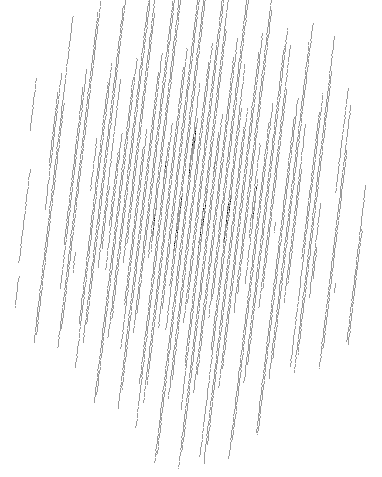# java.lang.Random falls "mainly in the planes"

The java.util.Random class uses an algorithm called a Linear Congruential Generator. As mathematics researcher George Marsaglia famously described1, one of the flaws of this algorithm is that the random numbers it generates "fall mainly in the planes". This essentially means that, among other flaws, a generator such as java.lang.Random is less suited to generating random tuples or combinations of different values.

### Falling in the planes

The problem manifests itself when we pull out series of numbers at a time from the generator. For reasons that will become clear, the problem is easiest to visualise with series of 3 numbers. Imagine we have a cube, which we will fill by plotting random points. We repeatedly take 3 numbers from our random nunmber generator, which we use for the x, y and z coordinates of the point. Doing this enough times, we'd eventually expect to fill the volume of the cube, given a couple of extra conditions2. But when we use a LCG, however large the period, what we actually find is that all of the points generated lie on a limited number of equally-spaced "planes" intersecting the cube. If you're not used to thinking mathematically about "planes", imagine a number of blades cutting through a block of cheese. Writing a computer program to plot our points on a cube, we end up with something such as the following:"Random" points plotted on a
cube using the infamous
RANDU algorithm.

Now, the seriousness of this problem depends on the parameters of the LCG (the values of a, c and m in the LCG equation introduced on the previous page). For illustration, we picked a particularly bad combination: the RANDU generator, something of a legend in how not to implement a random numbe rgenerator, and unfortunately used in various mainframes and scientific experiments until at least some time in the 1970s3. But even though this is pretty much the worst example there is, all LCG random number generators— including java.lang.Random— suffer from essentially the same problem, just on a smaller scale. With some slightly judicious mathematics, Marsaglia showed that the maximum number of planes depends on the modulus of the LCG (248 in the case of java.lang.Random, 231 in the case of RANDU), and on the number of dimensions. (The problem is easier to visualise in 2 or 3 dimensions, but mathematically, it extrapolates to any number of dimensions.) The bigger the modulus and the smaller the number of dimensions, the better:

maximum planes = (n! m) 1 / n

where n is the number of dimensions, and m is the modulus. (The ! is the factorial operator, so 3! is 1x2x3=6, 4! is 1x2x3x4=24 etc.) For Java's java.lang.Random modulus of 248, this gives a maximum of just over 119,000 planes4. This may not sound too bad, but it is something in the order of 0.003% of the theoretical maximum5.

Now you might be thinking, "well what the hell, I'm not using java.lang.Random to plot graphics". But see the illustration above as a graphical illustration of a mathematical problem: picking series of numbers from Random or any generator using the LCG algorithm will always introduce artefacts into the combinations of numbers produced. For example, generating strings of a certain length with successive calls to nextInt() will always produce strings with a subtle correlation between the characters in the string. Imagine then using these strings as test data for a hash table, and you might artificially get far more collisions than expected by chance, due to biases in the combinations of characters that the random number generator produced. So in general:

Avoid java.lang.Random (and any LCG) where you need to generate very random combinations of values. A common alternative in Java is ThreadLocalRandom.

Another significant flaw with LCGs is that the randomness of generated bits varies according to the bit position.